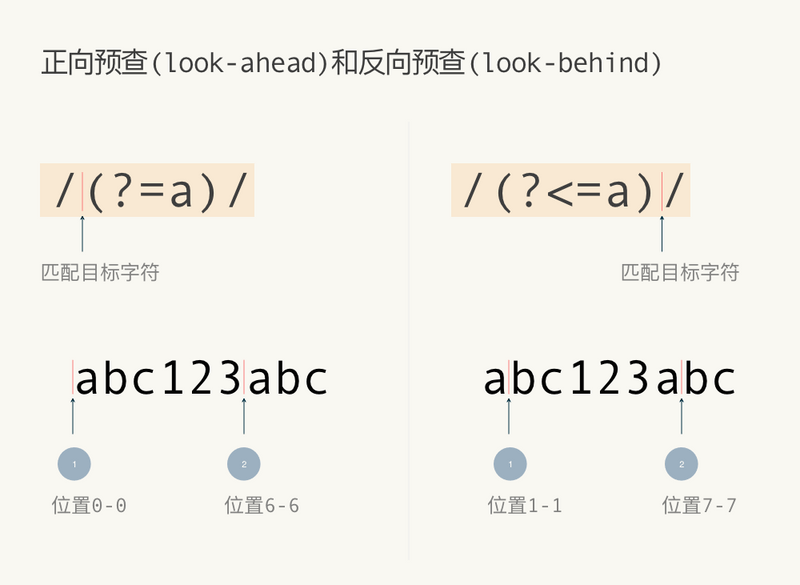# 正则里问号的作用

July 9, 2020 ... ☕️ 4 min read

## 问号的作用

### 量词

``````const reg = /\d+/;
const regLazy = /\d+?/;
const str = '123456';

str.match(reg); // 匹配到 123456
str.match(regLazy); 匹配到 1``````

``````x*?
x+?
x??
x{n}?
x{n,}?
x{n,m}?``````

### 断言MDN中的分组和预查的相关描述正向预查和反向预查

``````const str = 'bsabc大事情asd';
const reg = /(?<=a)s/;
str.match(reg); // 匹配到下标为9的s``````

``let reg = /^(?=.*[A-Z])(?=.*[a-z])(?=.*\d)[A-Za-z\d]{8,}\$/;``

### 分组

``'abc123def'.match(/(\d+)/); // 匹配组是123，groups是undefined``

``'abc111222def'.match(/(1+)(?:2+)/); // 匹配组是111，没有222，groups是undefined``

``'abc111222def'.match(/(?<TheChar>1+)(?:2+)/); // 匹配组是111，没有222，groups是groups: {TheChar: "111"}``
SideEffect is a blog for front-end web development.